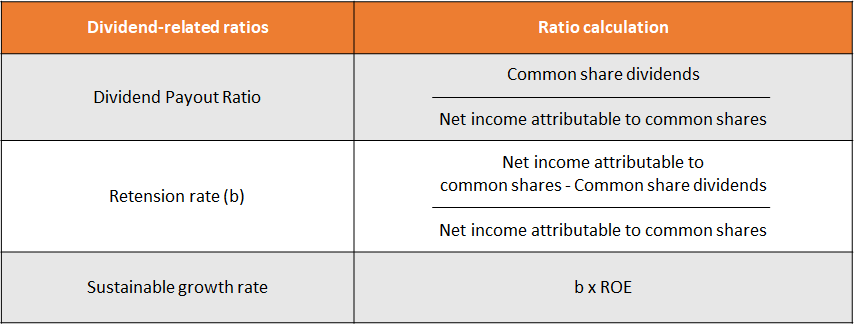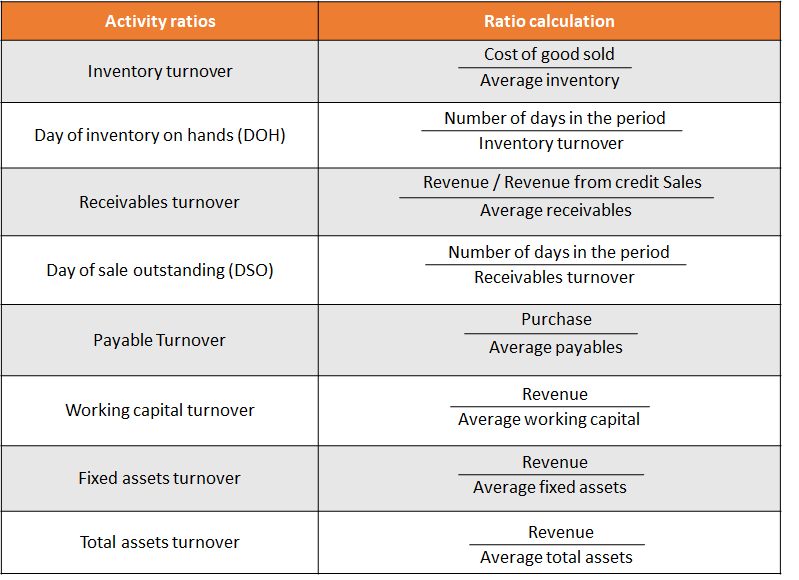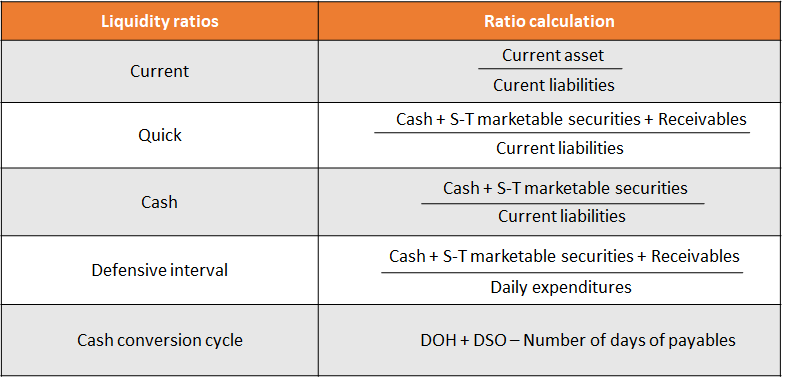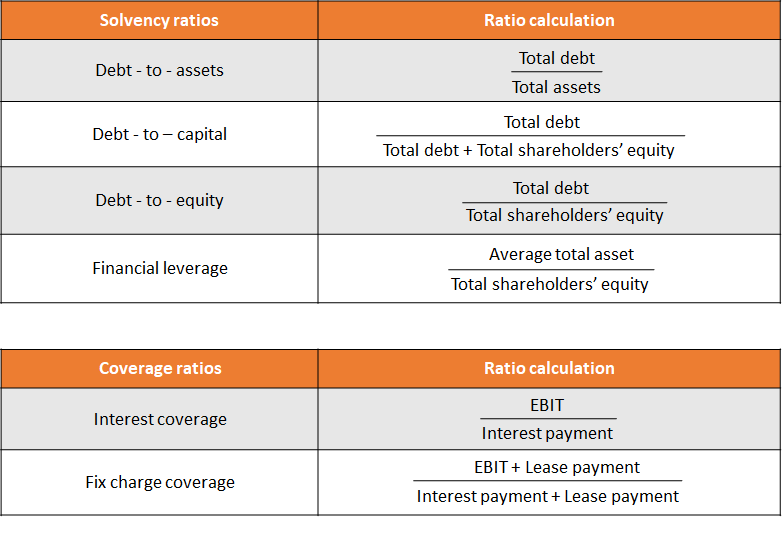# [FRA - Financial ratios ] - buổi 3

1. ROE, retention rateExample 1 :

1. A firm's financial statements reflect the following:

EBIT                      \$2,000,000

Sales                    \$16,000,000

Interest expense   \$900,000

Total assets           \$12,300,000

Equity                    \$7,000,000

Effective tax rate 35%

Dividend payout rate 28%

Cacular the firm's sustainable growth rate, tax burden, interest burden , EBIT margin ?

Growth rate          Tax burden          Interest burden         EBIT margin

A.      7.35%.                 0.65                       0.55                          0.125

B.      10.21%.               0.55                       0.65                          0.125

C.       7.35%                 0.5                         0.65                          0.125

Explanation

ROE = tax burden × interest burden × EBIT margin × asset turnover × financial leverage

tax burden = net income/EBT

EBT = EBIT - I = 2,000,000 - 900,000 = 1,100,000

NI = (EBT)(1-t) = (1,100,000)(1 - 0.35) = 715,000

Tax burden = NI/EBT = 715,000/1,100,000 = 0.65

interest burden = EBT/EBIT = 1,100,000/2,000,000 = 0.55

EBIT margin = EBIT/revenue = 2,000,000/16,000,000 = 0.125

asset turnover = revenue/total assets = 16,000,000/12,300,000 = 1.301

ROE = [(EBIT - I)(1-t)]/equity = [(2,000,000 - 900,000)(1 - 0.35)] / 7,000,000 0.1021

-> Sustainable growth  = ROE (1 - dividend payout rate)

= 0.1021 × 0.72 = 7.35%.

1.2. Would an increase in net profit margin or in the firm's dividend payout ratio increase a firm's sustainable growth rate?

Net profit margin       Dividend payout ratio

A.  Yes                  No

B.  Yes                  Yes

C.  No                    No

Explanation

The sustainable growth rate is equal to ROE multiplied by the retention rate. According to the Dupont formula, an increase in net profit margin will result in higher ROE. Thus, an increase in net profit margin will result in a higher growth rate. The retention rate is equal to 1 minus the dividend payout ratio. Thus, an increase in the dividend payout ratio will lower the retention rate and lower the growth rate

2. Activity Ratios

Activity ratios measure how efficiently a company performs day-to-day tasks, such as the collection of receivables and management of inventory.Example 2:

2. The following data applies to the XTC Company:

Sales             \$1,000,000.

Receivables   \$260,000.

Payables        \$600,000.

Purchases      \$800,000.

COGS            \$800,000.

Inventory        \$400,000.

Net Income     \$50,000.

Total Assets    \$800,000.

Debt/Equity = 200%.

What is the average collection period, the average inventory processing period, and the payables payment period for XTC  Company?

A.  45 days   45 days     132 days

B.  55 days   195 days    231 days

C.  95 days   183 days    274 days

Explanation

Receivables turnover = \$1,000,000 / \$260,000 = 3.840

Average collection period = 365 / 3.840 = 95.05 or 95 days

Inventory turnover = \$800,000 / \$400,000 = 2

Average inventory processing period = 365 / 2 = 183 days

Payables turnover ratio = \$800,000 / \$600,000 = 1.333

Payables payment period = 365 / 1.333 = 273.82 or 274 days

3. Liquidity Ratios

Liquidity ratios measure the company’s ability to meet its short-term obligations and how quickly assets are converted into cash.Example 3:

3. Johnson Corp. had the following financial results for the fiscal 2004 year:

Current ratio          2.00

Quick ratio             1.25

Current liabilities \$100,000

Inventory turnover  12

Gross profit            25%

The only current assets are cash, accounts receivable, and inventory. The balance in these accounts has remained constant throughout the year. Johnson's net sales for 2004 were:

A.   \$1,200,000.

B.   \$900,000.

C.   \$300,000.

Explanation

Gross profit = 1- COGS/Sales = 25% -> COGS is 75% of sales (1)

Current ratio = Current asset / Current liabilities

-> Current asset = 100,000*2 = \$200,000

Quick ratio = (Current asset - Inventory)/ Current liabilities

-> Inventory = \$75000

Inventory turnover = COGS/ Inventories

-> COGS = \$900,000 (2)

(1) & (2)  Sales = 900,000/75% = 1,200,000

3.1. A firm has a cash conversion cycle of 80 days. The firm's payables turnover goes from 11 to 12, what happens to the firm's cash conversion cycle? It:

A.  may shorten or lengthen.

B.  shortens.

C.  lengthens.

Explanation

CCC = collection period + Inv Period - Payment period.

Tăng vòng quay phải trả  làm giảm số ngày phải trả đi từ đó CCC dài hơn.

4. Solvency Ratios

Solvency ratios measure a company’s ability to meet long-term obligations. Subsets of these ratios are also known as “leverage” and “long-term debt” ratios.Example 4:

4.. Given the following income statement:

Net Sales                                200

Cost of Goods Sold                  55

Gross Profit                            145

Operating Expenses                30

Operating Profit (EBIT)           115

Interest                                     15

Earnings Before Taxes (EBT) 100

Taxes                                       40

Earnings After Taxes (EAT)      60

What are the interest coverage ratio and the net profit margin?

Interest Coverage Ratio         Net Profit Margin

A.    7.67                             0.30

B.    0.57                             0.56

C.    2.63                             0.30

Explanation

Interest coverage ratio = (EBIT / interest expense) = (115 / 15) = 7.67

Net profit margin = (net income / net sales) = (60 / 200) = 0.30

5. Profitability Ratios
Profitability ratios measure the company’s ability to generate profits from its resources (assets).Example 5:

5. McQueen Corporation prepared the following common-size income statement for the year ended December 31, 20X7:

Sales                           100%

Cost of goods sold      60%

Gross profit                  40%

For 20X7, McQueen sold 250 million units at a sales price of \$1 each. For 20X8, McQueen has decided to reduce its sales price by 10%. McQueen believes the price cut will double unit sales. The cost of each unit sold is expected to remain the same. Calculate the change in McQueen's expected gross profit for 20X8 assuming the price cut doubles sales.

A.  \$80 million increase.

B.  \$150 million increase.

C.  \$50 million increase.

Explanation

When sale reduce 10%, cost of each unit sold is expected to remain the same.

-> cogs/ 0.9 sales = 60%/0.9 = 67%

-> gross profit = 33%

change sale = 33% x 250 x 2 x 0.9 - 250 x1x40% = 48.5

5.1. Income Statements for Royal, Inc. for the years ended December 31, 20X0 and December 31, 20X1 were as follows (in \$ millions):

20X0                      20X1

Sales                                            78                          82

Cost of Goods Sold                     (47)                       (48)

Gross Profit                                   31                         34

Sales and Administration             (13)                       (14)

Operating Profit (EBIT)                  18                         20

Interest Expense                            (6)                       (10)

Earnings Before Taxes                  12                          10

Income Taxes                                 (5)                         (4)

Earnings after Taxes                       7                            6

Analysis of these statements for trends in operating profitability reveals that, with respect to Royal's gross profit margin and net profit margin:

A.  Gross profit margin decreased but net profit margin increased in 20X1.

B.  Gross profit margin increased in 20X1 but net profit margin decreased.

C.  Both gross profit margin and net profit margin increased in 20X1.